# Plotting in 3d

## Space curve

Example: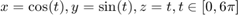t=linspace(0,6*pi);
x=cos(t); y=sin(t); z=t;
plot3(x,y,z)
axis equal; axis square;
grid on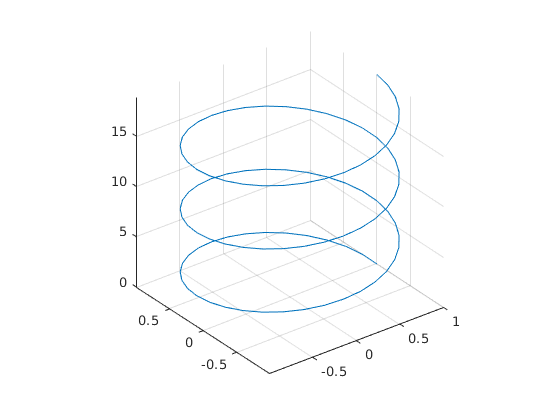## Another spacecurve

Hig-Hig p. 113-114

close all
t=-5*pi:.005:5*pi;
x=(1+t.^2).*cos(20*t);
y=(1+t.^2).*sin(20*t);
z=t;
plot3(x,y,z,'LineWidth',2)
grid on
FS='FontSize';
xlabel('x(t)',FS,14);ylabel('y(t)',FS,14)
zlabel('z(t)',FS,14,'Rotation',0,'HorizontalAlignment','right')
title('\it{plot3 example}',FS,14)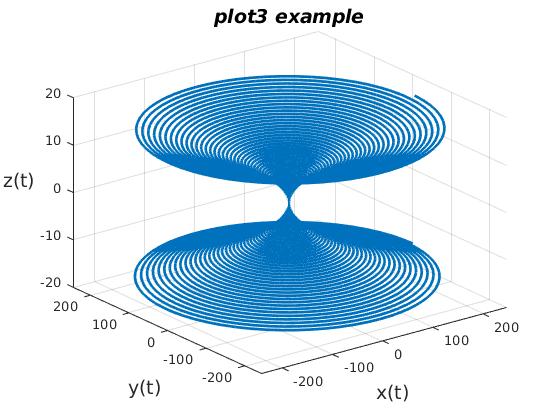## Surface plot, meshgrid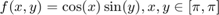x=linspace(-pi,pi,30);
y=x;     % No need to be the same.
% Now comes meshgrid:
[X,Y]=meshgrid(x,y);
Z=cos(X).*sin(Y);     % Remember: vector operation with (.)
% The data is ready, now "surf" it:
surf(x,y,Z),colorbar
% Several options, shading, colormap, m...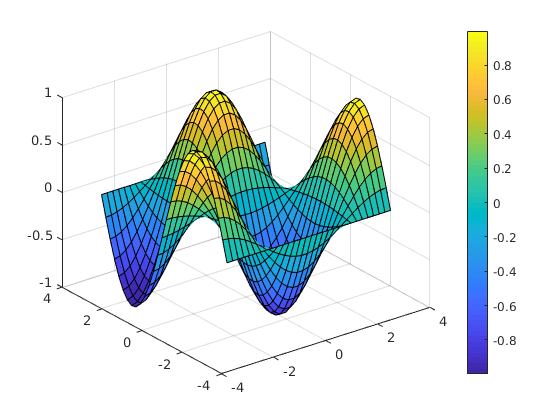## Contour plot

The same data is needed for contour plot, so we can continue:

contour(X,Y,Z,'LineWidth',2)
hold on
mesh(x,y,Z)
%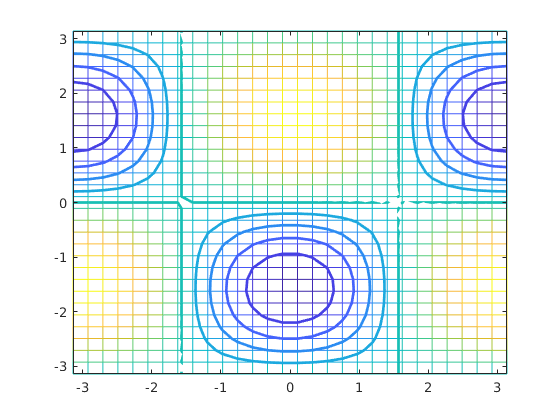## What does meshgrid do?

The Z-matrix could be obtained by

m=length(x);n=length(y);
for k=1:m
for l=1:n
Z(k,l)=cos(x(k))*sin(x(l));
end
end
hold off
mesh(x,y,Z)
% Well, this is at least tedious.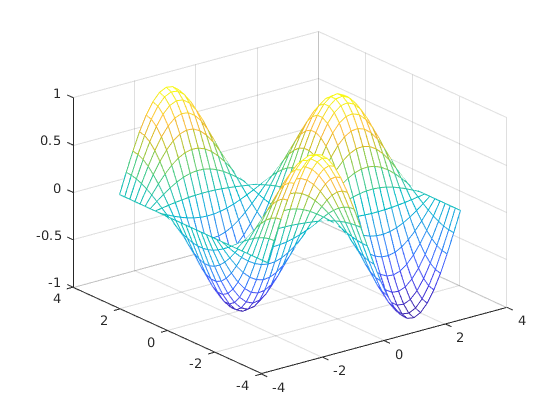## Lets look at small data:

x=0:3
y=-(0:2)
[X,Y]=meshgrid(x,y)
%
% X has duplicated rows, Y has duplicated columns
% X and Y are of same size
% Look at the points:
[X(:) Y(:)]
plot(X(:),Y(:),'*');shg
axis([-.5 3.5 -2.5 .5]);shg
figure
subplot(2,1,1)
imagesc(X) %,colorbar
title('upper X, lower Y')
subplot(2,1,2)
%title('Y')
imagesc(Y)

% All is right, isn't it!

x =

0     1     2     3

y =

0    -1    -2

X =

0     1     2     3
0     1     2     3
0     1     2     3

Y =

0     0     0     0
-1    -1    -1    -1
-2    -2    -2    -2

ans =

0     0
0    -1
0    -2
1     0
1    -1
1    -2
2     0
2    -1
2    -2
3     0
3    -1
3    -2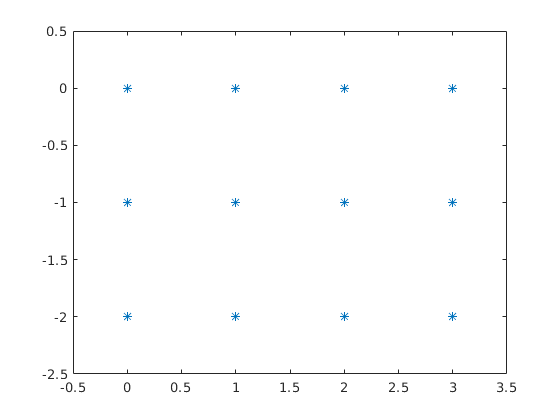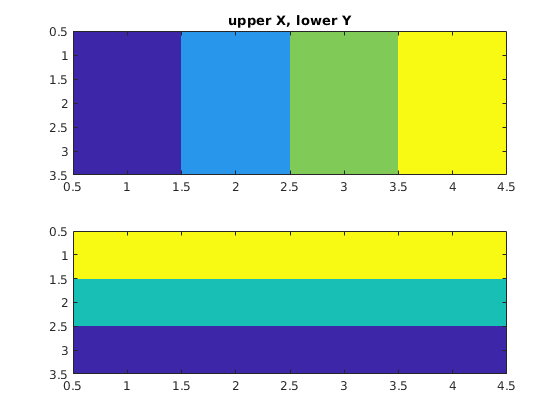## Example function plotsin2

This is an example of a function with no output argumrnts. type plotsin2 % From MIT-material plotsin(3,4)

plotsin(5)

## quiver-to add velocity vectors to a plot

This is a very nice function for making fieldplots to put arrows on points generated by meshgrid. Included in the materal of advanced course Spring 2018.